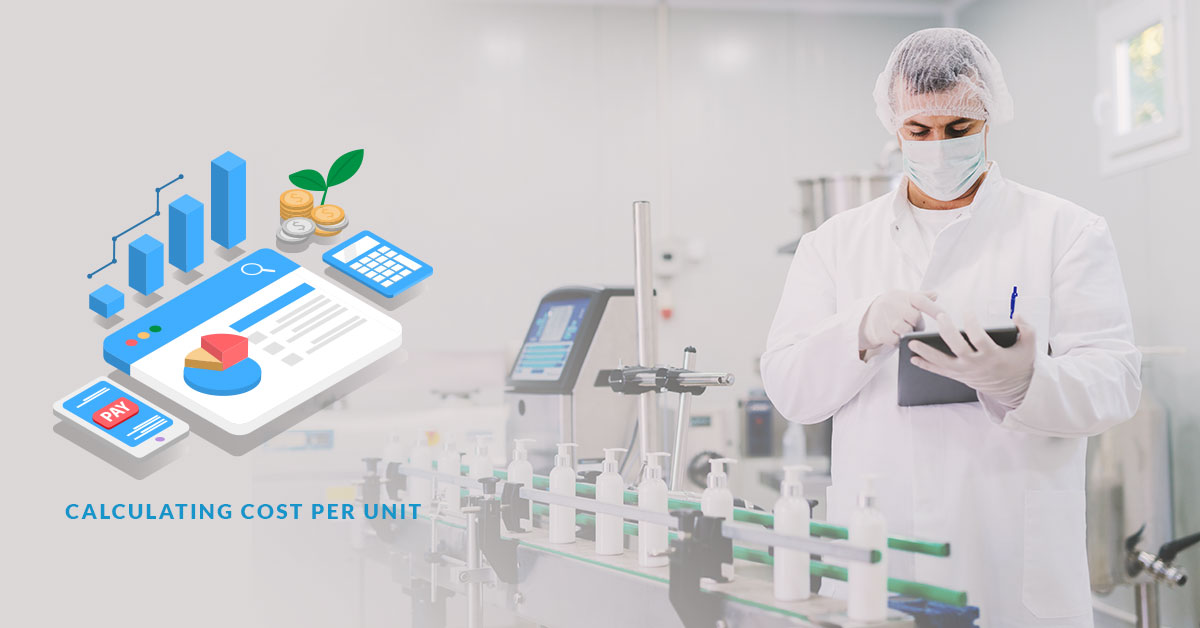# How to determine cost per unit for consumers (including import/export fees)

How to determine cost per unit for consumers (including import/export fees)
In order to calculate your fixed cost per unit there are several variables that need to be considered. However, those variables are also made up of a number of components.

The fixed cost per unit formula is fixed costs plus variable costs divided by the number of units produced. In mathematical terms: FC + VC / # of Units = Fixed Cost per Unit.

Fixed costs relate to premises, staffing, utilities and shipping, while variable costs relate to fluctuations in the cost of raw materials, foreign exchange costs and other non-fixed items.

Import and export costs can vary as the business becomes more experienced. With time you will make it possible to negotiate fixed contracts with transport and haulage firms or to even bring the operation in-house.

In order to be able to calculate your cost per item in advance or to be able to price your goods for end user or consumer pricing, one major consideration is the fluctuation in foreign exchange rates for the raw materials you are importing.

Depending upon your business you may be able to hedge some of your raw material purchase too but any business importing from overseas is running some foreign exchange exposure.It is prudent business practice to convert as many variable costs to fixed costs in order to be able to price your goods efficiently. To convert foreign exchange exposure from a variable to a fixed cost you will need to adopt a foreign exchange hedging policy. That policy is generally approved by your accountants or auditors in a note to the annual accounts.

For analysts to calculate selling price per unit from a firm’s income statement is a relatively simple matter which involves calculating the total cost of sales divided by the number of units sold. In order to determine cost per sale from a consumer price it is simply a matter of deducting the gross margin from 100% to give a percentage cost per sale. So, if you wish to make a gross markup of 40%, the cost is 60%. This method is used when discounting goods for either bulk sale or end of seasons.

The individual cost per sale formula is the product of volume of units sold divided by the sales price less gross margin (# of Units Sold/Sales Price – Gross Margin = Individual Cost per Sale).

To calculate the cost of goods available for sale, take the cost of inventory at the start of the year, add in purchases made that year plus cost of new production and that equates to the value of goods available for sale (cost of goods = inventory cost + purchases + new production) . Add in gross margin and divide by the number of units available to calculate sales price per unit.
(cost of goods + gross margin / units available for sale = sales price per unit)To calculate the standard cost per unit, divide total costs by number of units produced. If total costs are £5 million and you manufactured 1.5 million units, the simple cost per unit is £3.33 per unit.

Calculating and accounting for sales and costs can be as simple or as complicated as the firm wishes them to be. Continual reference to accountants is advisable because your simple goal is to sell as many units as possible of your product at a price that is both competitive and profitable. On the other side of the profit and loss account, costs need to be controlled as tightly as possible with variable converted to fixed whenever it is feasible.